## Percentage For SBI PO : Set – 15

1) The total number of students in a school is 5600 out of which 60% are boys what is the total number of girls in this school?

a) 2240

b) 3360

c) 2860

d) 3240

e) None of these

a)

Total number of students in a class=5600

Total number of boys = 60%

So total number of girls = 40%

40% of (5600) = 2240

2) If a man after spending 85% of the income he saves Rs. 4560 per month, his monthly income is

a) Rs. 32800

b) Rs. 31600

c) Rs. 30400

d) Rs. 29600

e) None of these

C)

Man spending income = 85%

So savings = 15%

15% of x = 4560

X= 30400

3) It is known that 6% of the mangoes are rotten. If the number of rotten mangoes is 54, then the total number of mangoes is

a) 900

b) 950

c) 1010

d) 1040

e) 1080

a)

6% of rotten mangoes are 54

So total number of mangoes = 6% of (x) =54

X = 900

4) A fruit seller had some oranges. He sells 35% oranges and still has 390 oranges. Originally he had

a) 620

b) 550

c) 600

d) 520

e) None of these

C)

Sold oranges = 35%

Total number of oranges = 100 – 35 = 65%

Remaining oranges =390

65% of X = 390

X=600

5) In an examination 90% of the students passed and 240 failed. How many students appeared for the examination?

a) 2360

b) 2400

c) 2450

d) 2520

e) 2580

b)

Percentage of passed students = 90%

Number of failed students = 240

10 % of x = 240

X = 2400

6) In a class of 60 students, each student got sweets that are 15% the total number of students. How many sweets were there?

a) 540

b) 520

c) 550

d) 580

e) 510

a)

Total number of students = 60

Each student got 15% of total number of students

So we get 15% of (60) = 9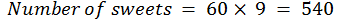7) In a class of 60 students and 6 teachers, each student got sweets that are 20% of the total number of students and each teacher got sweets that are 25% of the total number of students. How many sweets were there?

a) 875

b) 840

c) 810

d) 860

e) 895

c)

Number of sweets students got = 60*(20 % of (60)) = 720

Number of sweets Teachers got = 6 *(25% of (60)) = 90

Total number of sweets = 720 + 90 = 810

8) 720 sweets were distributed equally among children in such a way that number of sweets received by each child is 20% of the total number of children. How many sweets did each child receive?

a) 8

b) 10

c) 9

d) 12

e) None of these

d)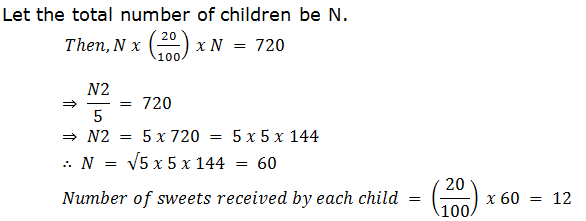9) A batsman scored 110 runs which included 3 boundaries and 8 sixes. What percent of his total score did he make by running between the wickets?

a) 45%

b) 54 6/11%

c) 55%

d) 48%

e) 45  5/11%

e)

Explanation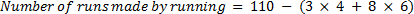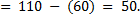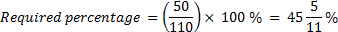10) The difference between a number and its two-fifth is 510. What is 10% of that number?

a) 12.75

b) 85

c) 204

d) 78

e) None of these